Newton's Third law of Motion

# Our Objective

To study the Third Law of Motion using two spring balances.

# The Theory

Newton’s Third Law of Motion states: ‘To every action there is an equal and opposite reaction’.

It must be remembered that action and reaction always act on different objects. The Third Law of Motion indicates that when one object exerts a force on another object, the second object instantaneously exerts a force back on the first object. These two forces are always equal in magnitude, but opposite in direction.

These forces act on different objects and so they do not cancel each other. Thus, Newton’s Third Law of Motion describes the relationship between the forces of interaction between two objects.

Whenever two bodies interact with each other, the force exerted by the first body on the second is called action. The force exerted by the second body on the first body is called reaction. The action and reaction are equal and opposite.

For example, when we placed a wooden block on the ground, this block exerts a force equal to its weight, W = mg acting downwards to the ground. This is the action force. The ground exerts an equal and opposite force W’ = mg on the block in the upward direction. This is the reaction force.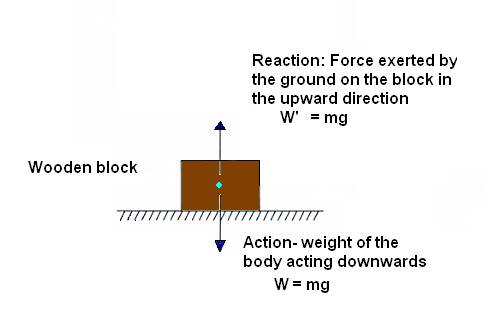## Applications of Newton’s Third Law of Motion

1.A gun recoils when a bullet is fired from it: When a bullet is fired from a gun, the gun exerts a force on the bullet in the forward direction. This is the action force. The bullet also exerts an equal force on the gun in the backward direction. This is the reaction force. Due to the large mass of the gun it moves only a little distance backward by giving a jerk at the shoulder of the gunman. The backward movement of the gun is called the recoil of the gun.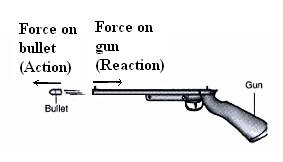2.A man walking on the ground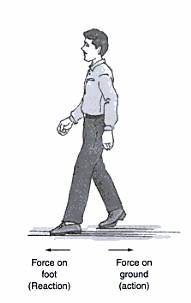3.Rowing a boat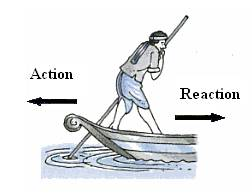4.A person is moving forward during swimming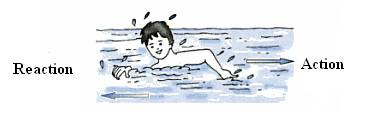5.Rocket propulsion# Learning Outcomes

The student will understand:

1. Action force
2. Reaction force
3. Newton’s Third Law of Motion.